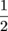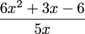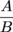Study Guide

# Rational Functions

## Rational Functions Introduction

Sometimes counting on all your fingers just won't cut it. Figuring out arithmetic calculations on your smartphone won't do the trick, either. When you've had it with figuring out simple stuff, like slicing and dicing a pizza into enough pieces to feed your army of deadbeat friends, check out rational functions.Some right-brain types would argue that this stuff is neither rational nor functional, but what do word nerds know?

You can do cool stuff with rational expressions. Say you're really into energy drinks. Sadly, you know they're oozing with all kinds of caloric nightmares—sugar, more sugar, and tons of sugar. How do you figure out how much your BMI (Body Mass Index) will increase if you pack in the sugar? You set up a rational function of the ratio between your height measurement and your weight. But we're getting ahead of ourselves. Let's get back to basics.

As a reminder, a rational number is a ratio of two integers:, for instance. Then we have the rational expression: the ratio of two polynomials.

"Poly...huh?" It sounds like something a parrot would say.

A polynomial is a numerical stew that contains variables, constants, and positive whole number exponents. A polynomial could be something like this: 6x2 + 3x – 6 (call it polynomial "A"). Another example of a polynomial is 5x (call it polynomial "B"). If we take the first polynomial and divide it by the second polynomial, we now have a numerator and a denominator. Now we have a rational expression that looks something like this:Generalizing the concept of rational expressions to its nuts and bolts, we can say that a rational expression can be written like this:...where A and B are both polynomials.

We can do great things with all this polynomial magnificence, except for one little thing. We can never, ever have a polynomial in the denominator that equals zero. No, the equation won't blow up, melt, burst into flames, or otherwise self-destruct if the denominator gets into the zero zone. But your work won't work. Trust us. In our little equation, 5x can never be zero. Just don't do it.

## Rational Functions Resources

### Websites

Rational Expressions
Rational expression galore—includes info on "asymptote-ing," graphing, and all that good stuff.

Direct and Inverse Variation
Practice makes perfect. You'll find lots of practice problems here to help you get your variation on.

Purplemath: Multiplying Rational Expressions
Need help with fractions? Simplifying? Factoring? It's all waiting for you here in purple-land.

Virtual Nerd: Solving Rational Equations
Don't worry—it's not too nerdy. This is a wicked-helpful site with excellent tutorials.

### Videos

How to Graph a Function With a Denominator
Here's a crazy video where the instructor writes backwards on a marker board. It's a must-see.

Solving Rational Equations
Watch and learn…you might just pick up something new about prying x outta those beastly fractions.

Simplify Complex Rational Expressions
If fractions still scare you, watch this.

### Games and Tools

Free Online Graphing Calculator
Graphing, but easier. Check out this free graphing calculator.

Free Math Solver
That's right—just type in the equation. It does all the work.

Cool Math Games
Take a math break. Have some fun! Strategy games, puzzles, logic, mazes…and even some pre-calculus lessons if you're really up for a challenge.